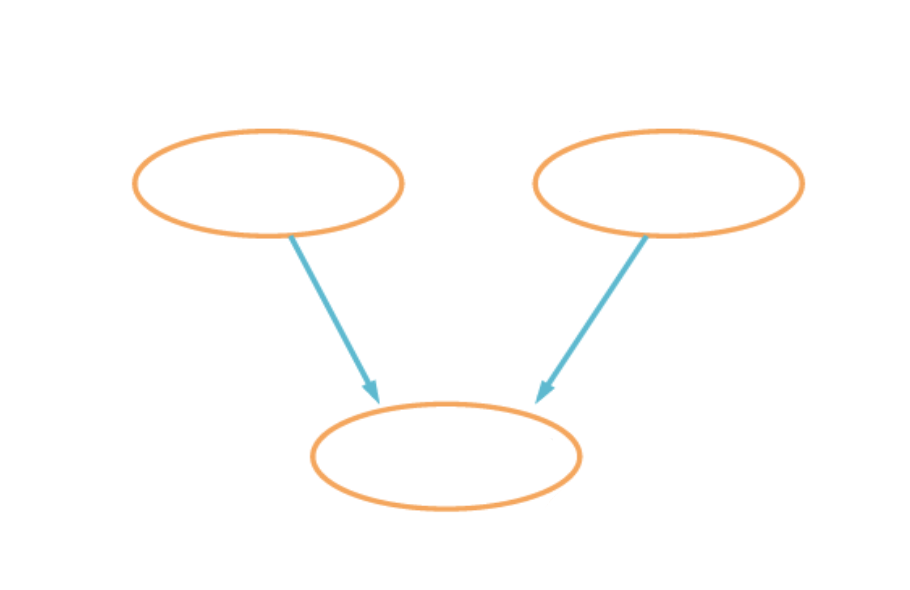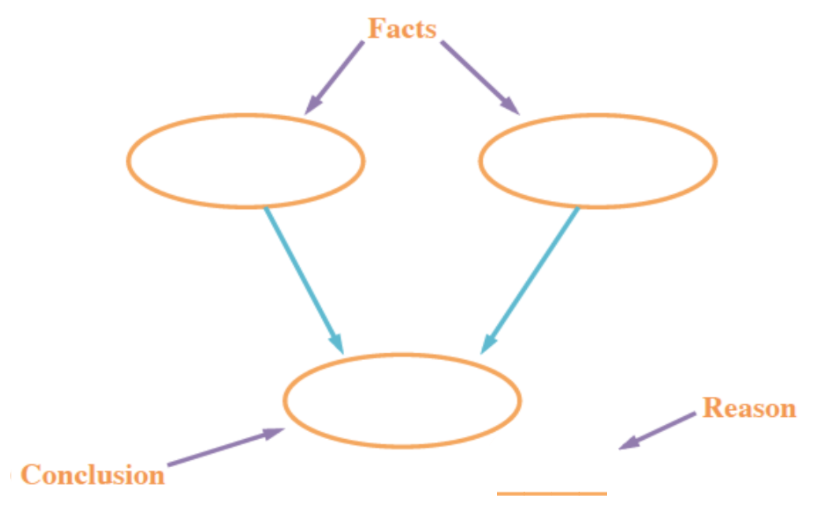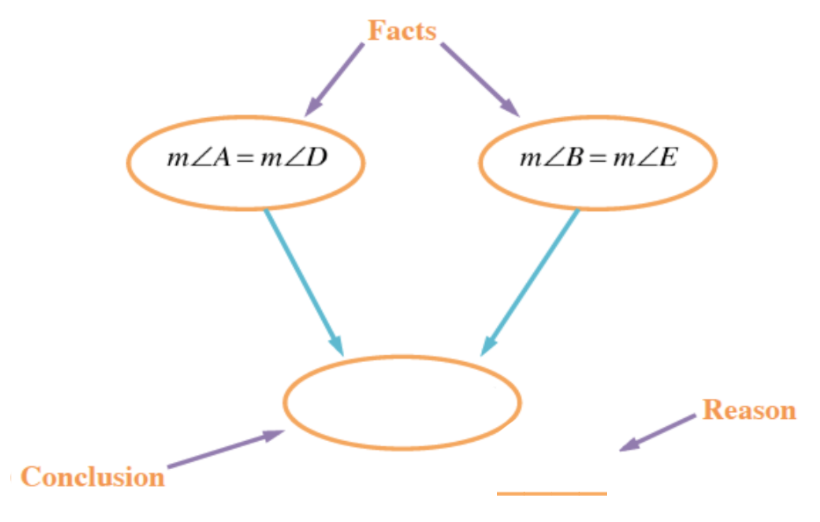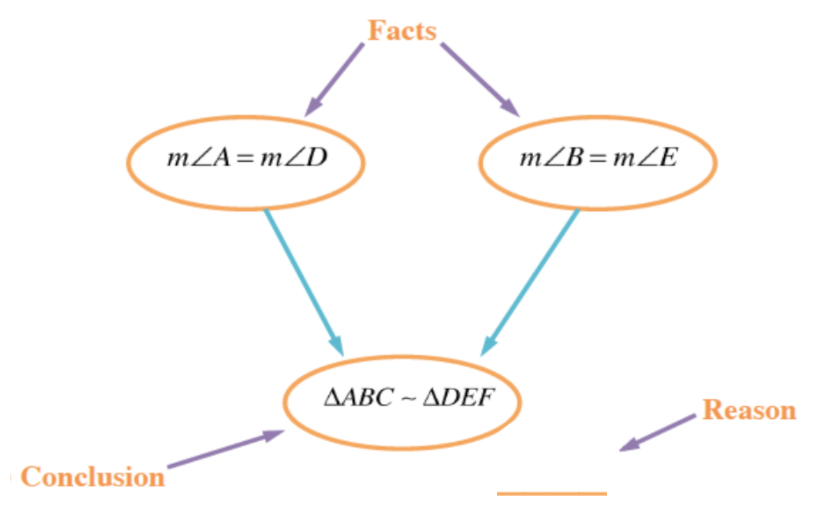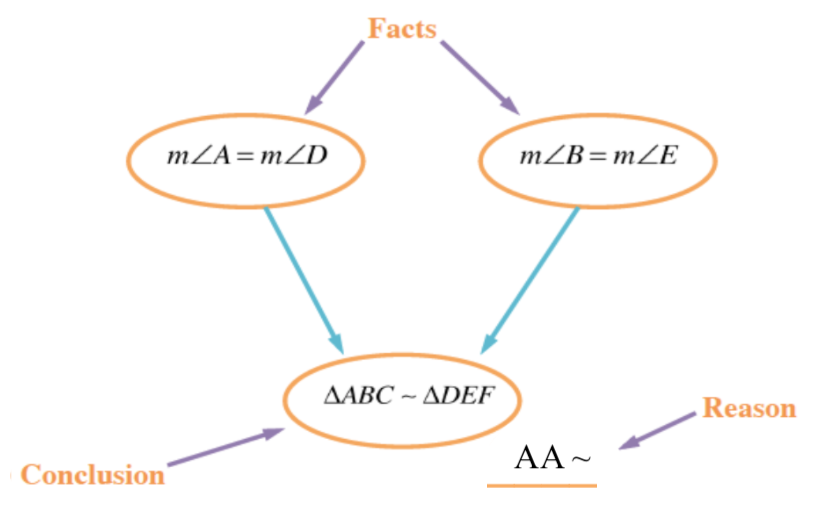### Home > GC > Chapter 4 > Lesson 4.1.1 > Problem4-7

4-7.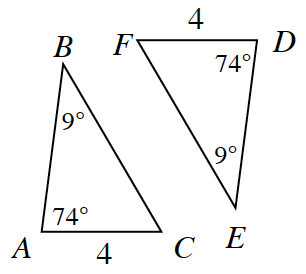Use the triangles below to answer the following questions.

1. Are the triangles above similar? How do you know? Show your reasoning in a flowchart.

2. Examine your work from part (a). Are the triangles also congruent? Explain why or why not.

First decide if the triangles are similar. Which triangle similarity condition did you use?

Draw the flowchart.

3 Ovals, Numbers 1 & 2 flow to #3.

Remember what belongs where in the construction of the flowchart.

Added: Blank line segment to the side & below oval #3, identified as Reason, Ovals 1 & 2 identified as facts, Oval 3 identified as Conclusion.

Fill in the flowchart. In the ''Facts'' ovals, write the facts that led you to the triangle similarity condition.

Labels added to oval #1, measure of angle A = measure of angle D, to oval #2, measure of angle b = measure of angle E.

In the ''Conclusion'' oval, write whether or not the triangles are similar.

Label added to oval #3, triangle, A B C is similar to triangle, D E F.

Below and to the right of the ''Conclusion'' oval, write the triangle similarity condition that you used.

Label added above Line segment, A A similarity.